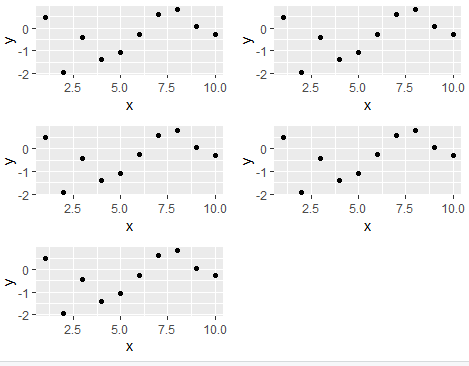3 views

library(ggplot2)

df <- data.frame(x=1:10, y=rnorm(10))

p1 <- ggplot(df, aes(x,y)) + geom_point()

plist <- list(p1,p1,p1,p1,p1)

# In my real example,a plot function will fit a ggplot to a list of datasets

#and return a list of ggplots like the example above.

I'd like to arrange the plots using grid.arrange() in gridExtra.

How would I do this if the number of plots in plist is variable?

This works:

grid.arrange(plist[],plist[],plist[],plist[],plist[])

but I need a more general solution. thoughts?

by

You can arrange a list of plots using grid.arrange as follows:

library(ggplot2)

df <- data.frame(x=1:10, y=rnorm(10))

p1 <- ggplot(df, aes(x,y)) + geom_point()

plist <- list(p1,p1,p1,p1,p1)

library(gridExtra)

n <- length(plist)

nCol <- floor(sqrt(n))

do.call("grid.arrange", c(plist, ncol=nCol))